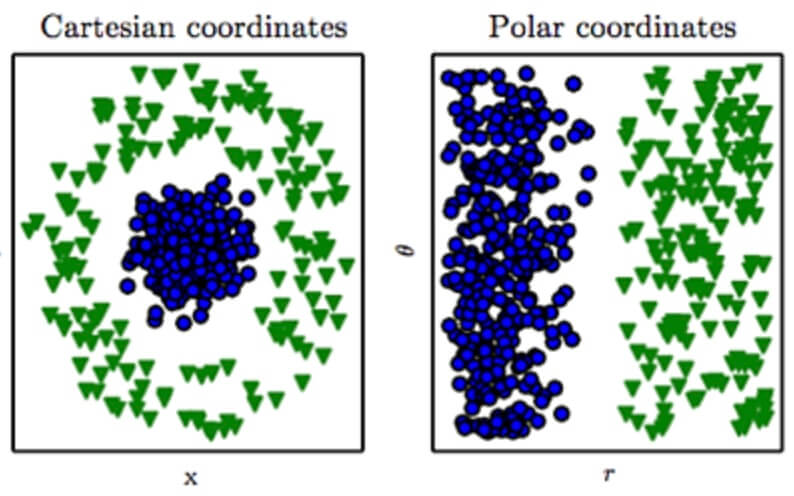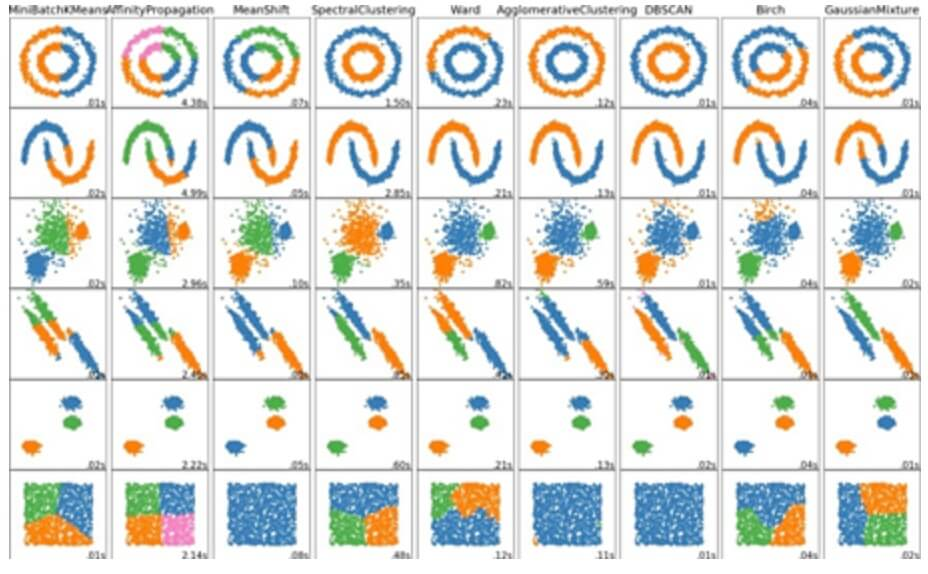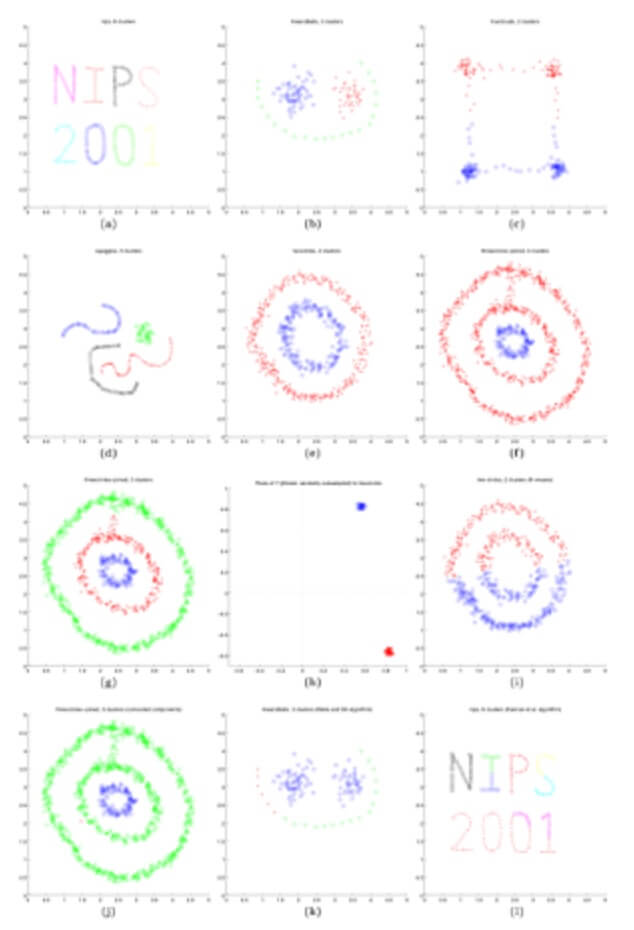# Choosing an Optimal Algorithm for AI in Cybersecurity

By
Sohrob Kazerounian
,
AI Research Lead at Vectra AI
and
|
August 15, 2018
Please note that this is an automated translation. For the most accurate information, refer to the original version in English.

In the last blog post, we alluded to the No-Free-Lunch (NFL) theorems for search and optimization. While NFL theorems are criminally misunderstood and misrepresented in the service of crude generalizations intended to make a point, I intend to deploy a crude NFL generalization to make just such a point.

You see, NFL theorems (roughly) state that given a universe of problem sets where an algorithm’s goal is to learn a function that maps a set of input data X to a set of target labels Y, for any subset of problems where algorithm A outperforms algorithm B, there will be a subset of problems where B outperforms A. In fact, averaging their results over the space of all possible problems, the performance of algorithms A and B will be the same.

With some hand waving, we can construct an NFL theorem for the cybersecurity domain: Over the set of all possible attack vectors that could be employed by a hacker, no single detection algorithm can outperform all others across the full spectrum of attacks.

## Optimally choosing an algorithm for AI in cybersecurity

At this point, you might be tempted to ask: If a given algorithm, on average, performs the same as all others in detecting the full breadth of attacks possible, why spend any effort developing machine learning (ML) algorithms for intrusion detection at all?

The reason, quite simply, is that NFL theorems (for search and optimization, and now cybersecurity) are only really applicable in a hypothetical world with a uniform prior over the space of all possible problems. In the real world, though, we only care about a small subset of these problems, and the prior over that subset of problems is not likely to be uniform. Add in the fact that any algorithm will be subject to space and runtime constraints as well as other real-world factors (e.g., the need for interpretability), and it quickly becomes clear that choices between algorithms can readily be measured against one another.

Nevertheless, while practical applications of NFL theorems don’t withstand technical rigor, they still serve as a good heuristic: No single algorithm will optimally meet the demands of a wide variety of problem types for which it could be deployed. This is evidenced by the fact that state-of-the-art techniques on tasks as varied as computer vision, natural language processing and financial prediction, all make use of different algorithms.

So how do we actually choose the best algorithm for cybersecurity?

Detecting wide-ranging attacker behaviors requires a suite of algorithms, each of which must be appropriately designed with a deep technical understanding of the potential algorithms that could be deployed and how they compare to one another, as well as integration of domain-specific knowledge necessary for optimal performance.

Each algorithm should be designed with the following questions in mind:

• Is the algorithm supervised or unsupervised? Regression or classification?
• Is there a natural space in which to represent or project inputs?
• If not, should the algorithm learn feature representations, or should inputs be used directly?
• Are inputs best represented as static or sequential/temporal data?
• If sequential, is it a classification or a labeling task?
• Are there temporal dependencies over a large number of timesteps? Alternatively, is the data simply Markovian?
• How much training data is there relative to the dimensionality of the input? Is the data (highly) non-linear?

## Domain knowledge

Algorithms require knowledge. Many off-the-shelf machine learning solutions can be deployed once basic questions about the nature of the problem are determined. Obtaining reliable results, however, requires a relatively deep mathematical understanding of the techniques in use, particularly as models become increasingly complex and intricate.

For example, consider the huge amount of interest in using a state-of-the-art neural network to process sequential and time-series data known as the long short-term memory (LSTM) model. While tools like TensorFlow, Google’s deep learning framework, tend to abstract the underlying math required to implement LSTM (in this case, a notation-heavy sequence of partial derivatives), knowledge is still required to use the algorithm correctly and for the right types of problems.

As in this case, many cybersecurity vendors tout their use of deep learning and LSTM models in particular. Yet, they incorrectly describe how this neural network actually functions and how they have applied it to threat detection. In addition to domain knowledge about algorithms, a deep understanding of the input domain can greatly simplify threat detection by downstream algorithms. As an example, consider a simple two-class classification task, where points that fall on a small circle are considered Class 0, and points that fall on a larger, surrounding circle are considered Class 1.

If the inputs are represented in Cartesian coordinates, no linear classifier will be able to learn to separate these two classes. If, instead, we know that the natural way to represent the inputs is not Cartesian coordinates but polar coordinates, the problem can easily be learned by a linear classifier. This is visualized in Fig. 1, where a linear decision boundary cannot be drawn in the Cartesian case but is trivial in the polar case.A simple two-class classification problem. When inputs are represented in a Cartesian coordinate system, no linear classifier can be learned to separate the two classes. In polar coordinates, however, the problem becomes trivial. Figure taken from "Deep Learning "Goodfellow et al. (2016)

## Anomaly detection vs. malicious behavior

In cybersecurity, anomaly detection is not the same thing as detecting malicious behavior.

Even if a statistical model accurately learns the underlying distributions of connection times, ports and other information a threat-detection system is supposed to track, a decision must be made about what constitutes normal behavior and what constitutes an outlier.

Let’s say we choose a threshold where any input that occurs with less than .01% chance is flagged as anomalous. Independent of the chosen statistical model, the more accurate the statistical model, the more likely we are to flag .01% of all inputs as outliers.

It is critical to avoid conflating outliers and anomalies with cyberattacks. Appropriate modeling of the various aspects of a cyberattack are requisite when trying to determine whether anomalous or outlier inputs are malicious.

Flagging anomalous or outlier data without considering the attack type can result in an attack being overlooked, because the input features that are tracked are not anomalous. Attacks can be modified to occur at normal times, on normal ports, from normal geolocations, and they would evade pure anomaly or outlier detection models.

## Unsupervised learning and dimension/manifold

Learning from unsupervised data is hard. Correctly learning from unsupervised data is even harder. In general, unsupervised learning is difficult due to a problem referred to as the curse of dimensionality.

This issue is that the dimensionality of the input data is often large enough that the volume of space it occupies grows at a much faster rate than the number of examples available to the algorithm.

This is true of data with a high number of dimensions or a large number of features, as well as time-series data that is often inherently high-dimensional. As such, unsupervised algorithms must often make inferences about the distributions data are drawn from, despite having insufficient evidence for the inferences they make.

Worse yet, even in situations where the input dimensionality is low, the specific manifolds on which data lie may not be conducive to specific learning techniques by different algorithms.

Imagine, for example, a dataset of two-dimensional inputs. Although the clusters seem obvious to the human eye, various unsupervised learning algorithms will attempt to model the data in multiple ways. Knowing which algorithms are appropriate for a given problem becomes increasingly pertinent.

The figure below shows a set of unsupervised learning algorithms and how they differ even in the most basic settings.Different unsupervised learning algorithms over a number of different data sets. The wide-ranging behavior of these algorithms, as shown by the variety of learned clusters, shows why an understanding of both the algorithm and the data is so critical.Figure taken from scikit-learn documentation.

Even within the same class of unsupervised learning algorithms, there may be many differing results. The following figure shows differences in learned clusters depending on the specific type of spectral clustering algorithm chosen.Results of different spectral clustering algorithms over a number or different datasets.Figure taken from "on Spectral Analysis and an Algorithm" [Ng, Jordan and Weiss (2001)]

Hopefully, the examples and descriptions in this entry have shown why it is so critical that a deep mathematical understanding of algorithms, coupled with an understanding of the cybersecurity domain in which the algorithm is to be applied, is necessary for constructing a performant IDS system.

There is no single algorithm that will outperform all the rest, nor is there a simple way to use algorithms “off-the-shelf.” There really is no free lunch.